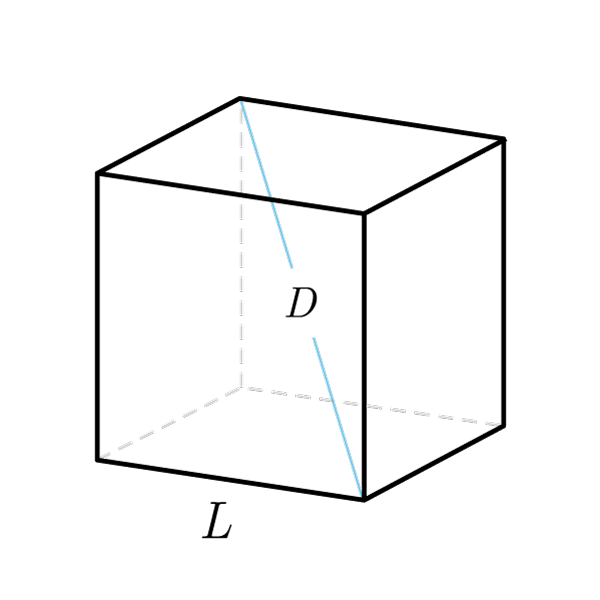# Cube

 Cube diagonal $$D$$ Side $$S$$ Base diagonal $$d$$ Base area $$A_{base}$$ Lateral surface $$S_{lat}$$ Total surface $$S_{tot}$$
$$V = {S}^3$$
Volume
$$S = \sqrt{V}$$
Edge
$$D = S\sqrt{3}$$
Cube diagonal
$$S = \frac{D}{\sqrt{3}}$$
Edge
$$A_{base} = {S}^{2}$$
Base area
$$S = \sqrt{A_{base}}$$
Edge
$$d = S\sqrt{2}$$
Base diagonal
$$S = \frac{d}{\sqrt{2}}$$
Edge
Total surface
$$S_{tot} = A_{base} + 2S_{lat}$$
Total surface
$$S_{tot} = 6{S}^{2}$$
Total surface
$$S_{tot} = 6A_{base}$$
Total surface
$$S = \sqrt{\frac{S_{tot}}{6}}$$
Edge
$$S_{tot} = 2{D}^{2}$$
Total surface
$$D = \sqrt{\frac{S_{tot}}{2}}$$
Cube diagonal
Lateral surface
$$S_{lat} = S_{tot} - 2A_{base}$$
Lateral surface
$$S_{lat} = 4{S}^{2}$$
Lateral surface
$$S = \sqrt{\frac{S_{lat}}{4}}$$
Edge
Cube inscribed into a sphere
$$R = \frac{\sqrt{3}}{2}S$$
$$S = \frac{2}{\sqrt{3}}R$$
Edge
Cube circumscribed to a sphere
$$r = \frac{S}{2}$$
$$S = 2r$$
Edge
$$R = r\sqrt{3}$$
$$r = \frac{R}{\sqrt{3}}$$

## Definition

A cube, or regular hexahedron, is a polyhedron bounded by 6 square faces, 8 vertices and 12 edges.

## Properties

1. 6 congruent square faces, perpendicular to two by two to each other
2. A cube is a regular hexahedron. A hexahedron is any polyhedron with six faces
3. All the  Square formulas are validCube Formulas
Data Formula
Volume V = S3
Edge S = 3√(V)
Cube diagonal D = S√3
Edge S = D / (√3)
Base area Abase = S2
Edge S = √(Abase)
Base diagonal d = S√2
Edge S = d / √2
Total surface Stot = Abase + 2 × Slat
Total surface Stot = 6S2
Total surface Stot = 6 × Abase
Edge S = √(Stot / 6)
Total surface Stot = 2D2
Cube diagonal D = √(Stot / 2)
Lateral surface Slat = Stot - 2 × Abase
Lateral surface Slat = 4S2
Edge S = √(Slat / 4)
Cube inscribed into a sphere
Data Formula
Circumscribed radius R = (√3/2) × S
Edge S = (2/√3) × R
Cube circumscribed to a sphere
Data Formula